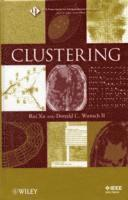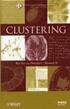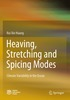Fler böcker inom
Format
Inbunden (Hardback)
Språk
Engelska
Antal sidor
368
Utgivningsdatum
2008-11-07
Upplaga
1
Förlag
John Wiley & Sons Inc
Medarbetare
Xu, Rui
Illustrationer
Illustrations
Dimensioner
236 x 163 x 23 mm
Vikt
636 g
Antal komponenter
1
Komponenter
52:B&W 6.14 x 9.21in or 234 x 156mm (Royal 8vo) Case Laminate on White w/Gloss Lam
ISBN
9780470276808

Du kanske gillarClustering

Inbunden Engelska, 2008-11-07
1379
• Skickas inom 7-10 vardagar.
• Gratis frakt inom Sverige över 159 kr för privatpersoner.
Finns även som
1649
This is the first book to take a truly comprehensive look at clustering. It begins with an introduction to cluster analysis and goes on to explore: proximity measures; hierarchical clustering; partition clustering; neural network-based clustering; kernel-based clustering; sequential data clustering; large-scale data clustering; data visualization and high-dimensional data clustering; and cluster validation. The authors assume no previous background in clustering and their generous inclusion of examples and references help make the subject matter comprehensible for readers of varying levels and backgrounds.

Passar bra ihop

1.2. +
3.De som köpt den här boken har ofta också köpt Heaving, Stretching and Spicing Modes av Rui Xin Huang (häftad).

Kundrecensioner

Har du läst boken? Sätt ditt betyg »

Fler böcker av författarna

• Handbook of Learning and Approximate Dynamic Programming

Jennie Si, Andrew G Barto, Warren B Powell, Don Wunsch

* A complete resource to Approximate Dynamic Programming (ADP), including on-line simulation code* Provides a tutorial that readers can use to start implementing the learning algorithms provided in the book* Includes ideas, directions, and recent ...

Recensioner i media

"This book provides a comprehensive and thorough presentation of this research area, describing some of the most important clustering algorithms proposed in research literature." ( Computing Reviews , June 2009) "The book covers a lot of ground in a relatively small number of pages, and should work well as a learning tool and reference." ( Computing Reviews , May 28, 2009)

Övrig information

Rui Xu , PhD, is a Research Associate in the Department of Electrical and Computer Engineering at Missouri University of Science and Technology. His research interests include computational intelligence, machine learning, data mining, neural networks, pattern classification, clustering, and bioinformatics. Dr. Xu is a member of the IEEE, the IEEE Computational Intelligence Society (CIS), and Sigma Xi. Donald C. Wunsch II , PhD, is the M.K. Finley Missouri Distinguished Professor at Missouri University of Science and Technology. His key contributions are in adaptive resonance and reinforcement learning hardware and applications, neurofuzzy regression, improved Traveling Salesman Problem heuristics, clustering, and bioinformatics. He is an IEEE Fellow, the 2005 International Neural Networks Society (INNS) President, and Senior Fellow of the INNS.

Innehållsförteckning

PREFACE. 1. CLUSTER ANALYSIS. 1.1. Classifi cation and Clustering. 1.2. Defi nition of Clusters. 1.3. Clustering Applications. 1.4. Literature of Clustering Algorithms. 1.5. Outline of the Book. 2. PROXIMITY MEASURES. 2.1. Introduction. 2.2. Feature Types and Measurement Levels. 2.3. Defi nition of Proximity Measures. 2.4. Proximity Measures for Continuous Variables. 2.5. Proximity Measures for Discrete Variables. 2.6. Proximity Measures for Mixed Variables. 2.7. Summary. 3. HIERARCHICAL CLUSTERING. 3.1. Introduction. 3.2. Agglomerative Hierarchical Clustering. 3.3. Divisive Hierarchical Clustering. 3.4. Recent Advances. 3.5. Applications. 3.6. Summary. 4. PARTITIONAL CLUSTERING. 4.1. Introduction. 4.2. Clustering Criteria. 4.3. K -Means Algorithm. 4.4. Mixture Density-Based Clustering. 4.5. Graph Theory-Based Clustering. 4.6. Fuzzy Clustering. 4.7. Search Techniques-Based Clustering Algorithms. 4.8. Applications. 4.9. Summary. 5. NEURAL NETWORK-BASED CLUSTERING. 5.1. Introduction. 5.2. Hard Competitive Learning Clustering. 5.3. Soft Competitive Learning Clustering. 5.4. Applications. 5.5. Summary. 6. KERNEL-BASED CLUSTERING. 6.1. Introduction. 6.2. Kernel Principal Component Analysis. 6.3. Squared-Error-Based Clustering with Kernel Functions. 6.4. Support Vector Clustering. 6.5. Applications. 6.6. Summary. 7. SEQUENTIAL DATA CLUSTERING. 7.1. Introduction. 7.2. Sequence Similarity. 7.3. Indirect Sequence Clustering. 7.4. Model-Based Sequence Clustering. 7.5. Applications-Genomic and Biological Sequence. 7.6. Summary. 8. LARGE-SCALE DATA CLUSTERING. 8.1. Introduction. 8.2. Random Sampling Methods. 8.3. Condensation-Based Methods. 8.4. Density-Based Methods. 8.5. Grid-Based Methods. 8.6. Divide and Conquer. 8.7. Incremental Clustering. 8.8. Applications. 8.9. Summary. 9. DATA VISUALIZATION AND HIGH-DIMENSIONAL DATA CLUSTERING. 9.1. Introduction. 9.2. Linear Projection Algorithms. 9.3. Nonlinear Projection Algorithms. 9.4. Projected and Subspace Clustering. 9.5. Applications. 9.6. Summary. 10. CLUSTER VALIDITY. 10.1. Introduction. 10.2. External Criteria. 10.3. Internal Criteria. 10.4. Relative Criteria. 10.5. Summary. 11. CONCLUDING REMARKS. PROBLEMS. REFERENCES. AUTHOR INDEX. SUBJECT INDEX.